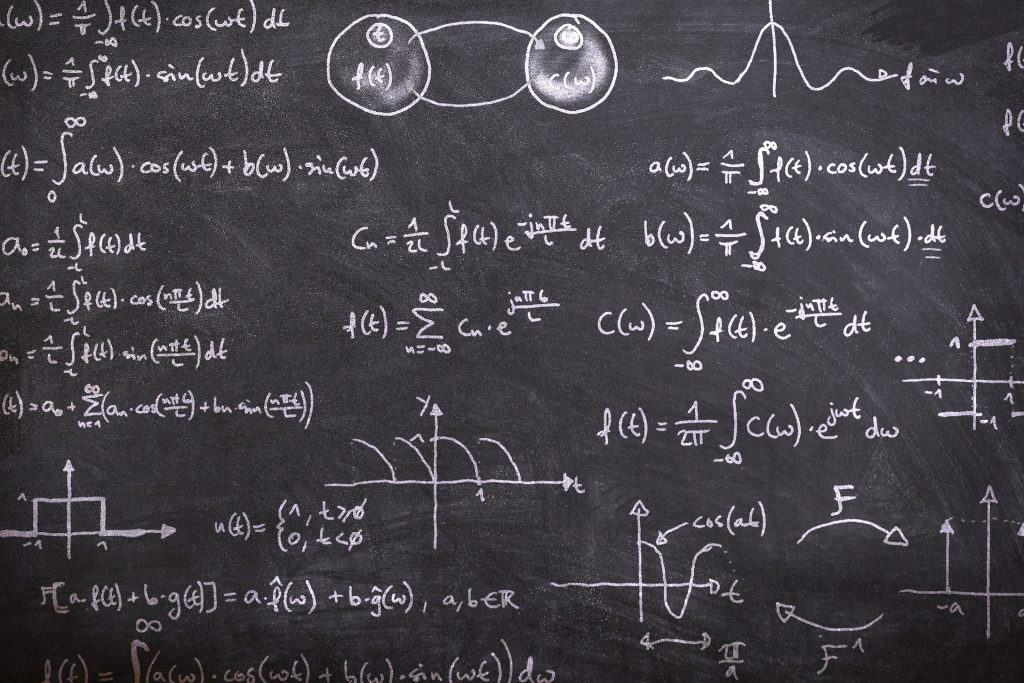Current Status
Not Enrolled
Price
Closed
Get Started
This course is currently closed

Welcome.

## Course Description

In this secondary 3 and 4 additional mathematics course, you will be learning

1.  Simultaneous Linear and Non-Linear Equations
3.  Surds and Indices
4.  Logarithmic and Exponential Functions
5.  Polynomials
6.  Partial Fractions
7.  Binomial Theorem
8.  Coordinate Geometry
9.  Linear Law
10.  Circles
11.  Trigonometric Functions

1.  Simple Trigonometric Identities and Equations
2.  Further Trigonometric Identities
3.  Differentiation
4.  Equations of Tangent and Normal
5.  Rates of Change
6.  Maxima and Minima Problems
7.  Further Differentiation
8.  Integration
9.  Further Integration
10.  Applications of Integration
11.  Kinematics
12.  Plane Geometry

## Course Content

Lesson Content
0% Complete 0/9 Steps
Lesson Content
0% Complete 0/30 Steps
Lesson Content
0% Complete 0/44 Steps
Lesson Content
0% Complete 0/19 Steps
Lesson Content
0% Complete 0/11 Steps
Lesson Content
0% Complete 0/12 Steps
Lesson Content
0% Complete 0/10 Steps
Lesson Content
0% Complete 0/9 Steps
Lesson Content
0% Complete 0/3 Steps
Lesson Content
0% Complete 0/28 Steps
Lesson Content
0% Complete 0/11 Steps
Lesson Content
0% Complete 0/18 Steps
Lesson Content
0% Complete 0/29 Steps
Lesson Content
0% Complete 0/5 Steps
Lesson Content
0% Complete 0/7 Steps
Lesson Content
0% Complete 0/18 Steps
Lesson Content
0% Complete 0/41 Steps
Lesson Content
0% Complete 0/31 Steps
Lesson Content
0% Complete 0/35 Steps
Lesson Content
0% Complete 0/17 Steps
Lesson Content
0% Complete 0/14 Steps
Lesson Content
0% Complete 0/4 Steps